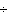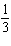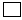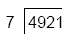Multiple Choice
Identify the choice that best completes the statement or answers the question.

1.

Computation – Decimals – RIT 221 – 230

Sally earned 3/4 of a dollar. How much money was that?
 a. \$0.10 d. \$0.30 b. \$0.25 e. \$0.45 c. \$0.75

2.

4,439 - 26.35 =
 a. 1,804 d. 6,674 b. 4,412.65 e. 7,704 c. 4,412.35

3.

Choose the number sentence that best relates to the problem. Do not
compute. The seventh and eighth graders at Midville Middle School held a fundraiser. The seventh graders raised \$526.34 and the eighth graders raised \$601.87.
How much more money did the eighth graders raise than the seventh graders?
 a. \$601.87 + \$526.34 = d. \$526.34 - \$601.87 = b. \$526.34 + \$601.87 = e. \$70 c. \$601.87 - \$526.34  =

4.

Convert 5/8 to a decimal.
 a. 0.652 d. 0.58 b. 0.8 e. 0.625 c. 0.5

5.

Subtract using a calculator.
0.862 - 0.34967 =
 a. 0.51267 d. 1.21167 b. 0.34105 e. 0.63491 c. 0.51233

6.

3.627 x 100 =
 a. 0.03627 d. 362.7 b. 0.3627 e. 3,627 c. 36.27

7.

300,003 =
 a. 0.001 d. 100 b. 0.1 e. 10,000 c. 10

8.

Computation – Fractions – RIT 221 – 230

7/8 + 6/8 =
 a. 13/16 d. 1 5/8 b. 7/4 e. 1 5/16 c. 13/64

9.

Write in simplest form.
16/36
 a. 1/2 d. 4/9 b. 4/6 e. 32/72 c. 8/18

10.

Give the answer in lowest terms.
9
15
1
4

 a. A. 22/60 d. 1/3 b. 8/11 e. 10/19 c. 7/20

11.

7
-  2 3/5
 a. 4 2/5 d. 6/5 b. 5 3/5 e. 5 2/5 c. 9 2/5

12.

Estimate. Do not compute.
5 6/7 - 2 1/9 =
 a. 9 d. 2 b. 7 e. 4 c. 3

13.

43=
 a. 1 4/21 d. 1 13/35 b. 106/70 e. 1 26/70 c. 2

14.

Computation – Integers – RIT 221 – 230

- 240(-6)  = ___
 a. 4 d. –4 b. 40 e. 400 c. –40

15.

Computation – Percent – RIT 221 – 230

Find the percent.
Bill correctly answered 78 out of 100 problems on his test. What percent did
he get correct?
 a. 78% d. 70% b. 22% e. .78% c. 7.8%

16.

15 is what % of 60?
 a. 15/60% d. 25% b. 15% e. 4% c. 60%

17.

10 is 50% of what number?
 a. 5 d. 20 b. 100 e. 200 c. 50

18.

Computation – Whole Numbers – RIT 221 – 230

30 +=  97 + 10
 a. 67 d. 97 b. 107 e. 10 c. 77

19.a. 73 d. 730 b. 703 e. 63 c. 81

20.

Divide using a calculator.  1716 ¸ 52 =
 a. 56 d. 40 R2 b. 33 R8 e. 33 c. 36

21.

Which number is compatible with 240?
 a. 21 d. 351 b. 7 e. 62 c. 8